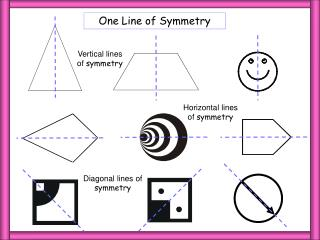DownloadDownload Presentation1 LoS

# 1 LoS

Télécharger la présentation## 1 LoS

- - - - - - - - - - - - - - - - - - - - - - - - - - - E N D - - - - - - - - - - - - - - - - - - - - - - - - - - -
##### Presentation Transcript

1. 1 LoS One Line of Symmetry Vertical lines of symmetry Horizontal lines of symmetry Diagonal lines of symmetry

2. 2 LoS 2 Lines of Symmetry

3. Rectangle Mirror Line Half a rectangle The Rectangle Problem A rectangle has only 2 lines of symmetry and not 4 like the square  To see this consider the following:

4. The Rectangle Problem A rectangle has only 2 lines of symmetry and not 4 like the square  To see this consider the following: A reflection in the diagonal would produce a kite! Mirror Line Half a rectangle

5. 3 LoS 3 Lines of Symmetry

6. 4 LoS 4 Lines of Symmetry

7. 5 LoS 5 Lines of Symmetry

8. 6 LoS 6 Lines of Symmetry

9. Regular Regular Polygons Equilateral Triangle Square Regular Pentagon Regular polygons have lines of symmetry equal to the number of sides/angles that they possess. Regular Octagon Regular Hexagon

10. Mix 1 How many lines of symmetry for each shape? 6 4 3 5 8

11. Mix 2 How many lines of symmetry for each shape? 3 6 5 4 5

12. Mix 3 How many lines of symmetry for each shape? 3 2 5 2 4

13. Mix 4 How many lines of symmetry for each shape? 6 2 4 2 1 1

14. Mix 5 How many lines of symmetry for each shape? 1 2 4 3 5

15. Mix 6 How many lines of symmetry for each shape? 8 1 4 1 6

16. Plane Symmetry

17. Plane Symmetry A plane of symmetry divides a three dimensional shape into two congruent halves that are mirror images of each other. A Cuboid A Cuboid has 3 planes of symmetry

18. Plane Symmetry A plane of symmetry divides a three dimensional shape into two congruent halves that are mirror images of each other. A Cuboid A Cuboid has 3 planes of symmetry

19. Plane Symmetry A plane of symmetry divides a three dimensional shape into two congruent halves that are mirror images of each other. A Cuboid A Cuboid has 3 planes of symmetry

20. Cuboid 3 Planes of symmetry

21. No Diagonal Can you explain why the plane shown is not a plane of symmetry? This is similar to the situation for the rectangle which does not have a line of symmetry through its diagonal. Reflection through the diagonal produces a kite.

22. Plane Symmetry A plane of symmetry divides a three dimensional shape into two congruent halves that are mirror images of each other. A square based prism A square based prism has 5 Planes of symmetry

23. Plane Symmetry A plane of symmetry divides a three dimensional shape into two congruent halves that are mirror images of each other. A square based prism A square based prism has 5 Planes of symmetry

24. Plane Symmetry A plane of symmetry divides a three dimensional shape into two congruent halves that are mirror images of each other. A square based prism A square based prism has 5 Planes of symmetry

25. Plane Symmetry A plane of symmetry divides a three dimensional shape into two congruent halves that are mirror images of each other. A square based prism A square based prism has 5 Planes of symmetry

26. Plane Symmetry A plane of symmetry divides a three dimensional shape into two congruent halves that are mirror images of each other. A square based prism A square based prism has 5 Planes of symmetry

27. 5 Planes of symmetry Square based prism

28. Cube The 9 Plane Symmetries of the Cube

29. Triangular Isos Triangular Based Prisms An isosceles triangular based prism has 2 planes of symmetry.

30. Triangular Based Prisms An isosceles triangular based prism has 2 planes of symmetry.

31. Triangular Based Prisms An isosceles triangular based prism has 2 planes of symmetry.

32. Triangular Equilateral Triangular Based Prisms An equilateral triangular based prism has four planes of symmetry.

33. Triangular Based Prisms An equilateral triangular based prism has four planes of symmetry.

34. Triangular Based Prisms An equilateral triangular based prism has four planes of symmetry.

35. Triangular Based Prisms An equilateral triangular based prism has four planes of symmetry.

36. Triangular Based Prisms An equilateral triangular based prism has four planes of symmetry.

37. Pyramids Pyramids A rectangular based pyramid has 2 planes of symmetry.

38. Pyramids A square based pyramid has 4 planes of symmetry.

39. Pyramids Regular Tetrahedron: 6 planes of symmetry

40. Questions State the number of planes of symmetry for each shape 1 2 3 6 2 1 4 5 6 Infinite 1 5 8 9 7 2 3 4